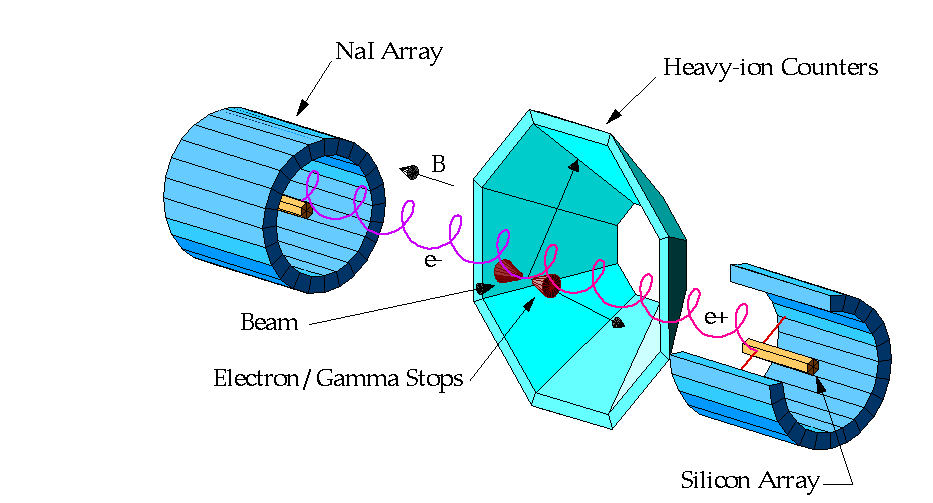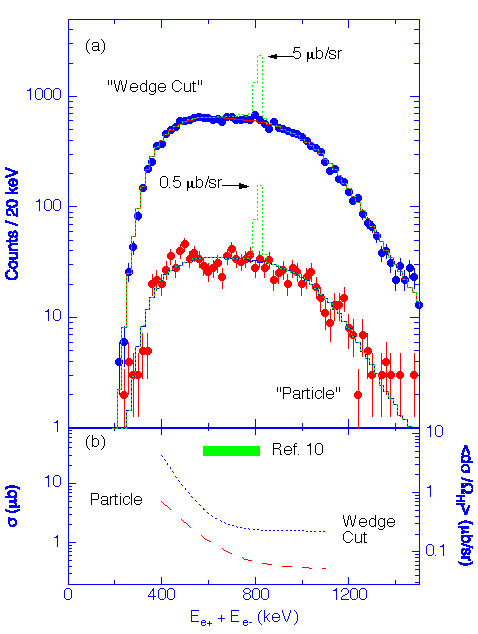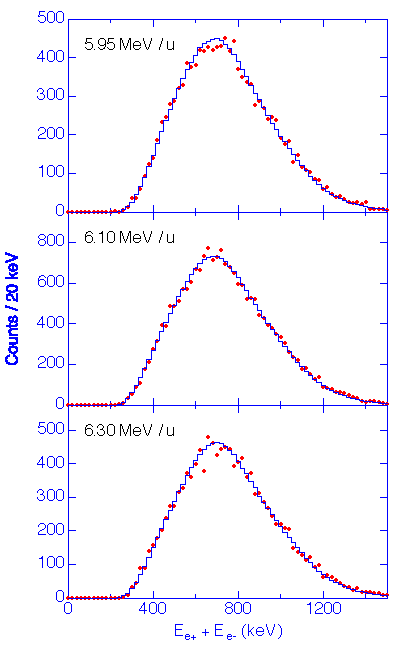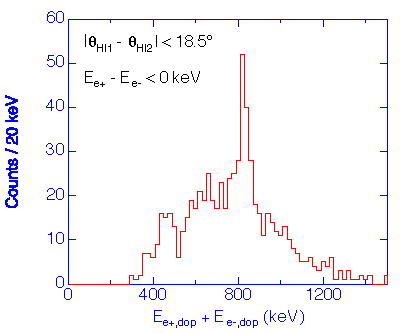A NEW LOOK AT POSITRON PRODUCTION FROM HEAVY-ION COLLISIONS: RESULTS FROM APEX +

I.Ahmad,[a] Sam.M.Austin,[b] B.B.Back,[a] R.R.Betts,[a,c[ F.P.Calaprice,[d] K.C.Chan,[e] A.Chishti,[e] P.Chowdhury,[e] C.Conner,[c] R.W.Dunford,[a] J.D.Fox,[f] S.J.Freedman,[a,g] M.Freer,[a] S.B.Gazes,[h,i] A.L.Hallin,[j] Th.Happ,[a,k] D.Henderson,[a] N.I.Kaloskamis,[e] E.Kashy,[b] W.Kutschera,[a] J.Last,[a] C.J.Lister,[a] M.Liu,[j] M.R.Maier,[g] D.M.Mercer,[b] D.Mikolas,[b] P.A.A.Perera,[h] M.D.Rhein,[a,k] D.E.Roa,[f] J.P.Schiffer,[a,i] T.A.Trainor,[l] P.Wilt,[a] J.S.Winfield,[b] M.Wolanski,[a,i] F.L.H.Wolfs,[h] A.H.Wuosmaa,[a] G.Xu,[e] A.R.Young,[d] and J.E.Yurkon[b]

[a] Physics Division, Argonne National Laboratory, Argonne, IL60439, USA

[b] NSCL and Department of Physics&Astronomy, Michigan State University, East Lansing, MI48824, USA

[c] Physics Department, University of Illinois at Chicago, Chicago, IL60607, USA

[d] Physics Department, Princeton University, Princeton, NJ08544, USA

[e] A.W.Wright Nuclear Structure Laboratory, Yale University, New Haven, CT06511, USA

[f] Physics Department, Florida State University, Tallahassee, FL32306, USA

[g] Lawrence Berkeley Laboratory, Berkeley, CA94720, USA

[h] Nuclear Structure Research Laboratory, University of Rochester, Rochester, NY14627, USA

[i] Department of Physics, University of Chicago, Chicago, IL60637, USA

[j] Physics Department, Queen's University, Kingston, Ontario, K7L 3N6, Canada

[k] Gesellschaft für Schwerionenforschung, Darmstadt, Germany

[l] Nuclear Physics Laboratory, University of Washington, Seattle, WA98195, USA

Presented by F. L. H. Wolfs

Abstract

A new solenoidal spectrometer, designed to study the production mechanism of electrons and positrons in heavy-ion collisions, has been constructed at Argonne National Laboratory. The spectrometer has been used to study the 238U + 181Ta system at 5.95, 6.10, and 6.30 MeV/u and the 238U + 232Th system at 5.95 MeV/u. These bombarding energies cover the energy region where previous experiments have reported sharp sum-energy lines. No evidence is found for sharp peaks in the present data. For the specific case of the isolated decay of a neutral particle of mass 1.4 - 2.1 MeV/c2 the upper limits on cross sections obtained from the present data are significantly less than the previously reported cross sections.

I. Introduction

The peaks observed in the energy spectra of positrons emitted in certain heavy-ion collisions at energies close to the Coulomb barrier are a surprising and unexpected result for which there has been no consistent explanation despite more than 10 years of research. The peaks were observed in a number of experiments [1-4] carried out using two different spectrometers. Subsequent experiments showed that, for some systems, similar structures existed in the energy spectra of electrons detected in coincidence with positrons and that the laboratory sum-energy spectra of positrons and coincident electrons also showed sharp lines [5-12]. The observation that the lines in the sum-energy spectra were narrower than those in the singles spectra and the near equality of the positron and electron energies suggested that the origin of the peaks, in some cases, might be the two-body decay of an isolated, slow-moving neutral object. The lack of direct information on the lepton emission angles, however, precluded proof of this hypothesis.

A new spectrometer, designed to study the production mechanism of electrons and positrons in heavy-ion collisions, has been constructed at the ATLAS Accelerator Facility at Argonne National Laboratory [13-15]. The potential data rate capability of the ATLAS Positron EXperiment (APEX) is over an order of magnitude larger than those of the previously published experiments, due in part to an increase of detection efficiency, but mainly as a result of the continuous-wave nature of the heavy-ion beams from the ATLAS accelerator. In addition, APEX measures the angles of emission of the electrons and positrons, thus allowing a direct determination of the kinematics of the positron-electron pairs.

In this paper we present the first results obtained with APEX. Data were obtained for the 238U + 181Ta system at 5.95, 6.10, and 6.30 MeV/u and for the 238U + 232Th system at 5.95 MeV/u. These results are discussed in more detail in Ref. .Fig.1. Schematic drawing of APEX showing the major components. For reasons of clarity, the various monitor detectors are not shown.

II. Principle of Operation

APEX consists of a large solenoidal spectrometer, mounted transverse to the beam direction as shown schematically in Figure 1. Electrons and positrons produced at the target position follow helical trajectories in the 300 Gauss magnetic field and are detected, close to the solenoid axis, by two highly segmented, 33 cm long, 3 cm diameter, pencil-like arrays of silicon detectors each consisting of 198, 1 mm thick PIN diodes. The angles of emission of the leptons are determined from their detected position, obtained from the segmentation of the silicon array, and their measured time-of-flight and energy. Positrons which stop in the silicon detectors are distinguished from electrons by detecting their annihilation photons in cylindrical arrays of position-sensitive NaI(Tl) detectors that surround the silicon arrays. Scattered heavy-ions are detected in coincidence in an array of heavy-ion detectors that cover the angular range between 20deg. and 68deg. with respect to the beam direction. Two conical heavymet shields ("electron/gamma stops") are positioned on the solenoid axis on either side of the target in order to shield the NaI(Tl) and silicon arrays from the direct flux of primary [[gamma]]-rays, and to prevent electrons with energies below 115 keV from reaching the silicon arrays. Additional detectors are provided to monitor the beam intensity, position, and timing, as well as the target condition. The apparatus and its components are described in detail in Ref. .

III. Lepton Performance

The performance of the APEX spectrometer for electrons and positrons has been studied extensively with radioactive sources and various in-beam measurements. The overall performance of the silicon arrays is illustrated in Figure 2a which shows an energy spectrum obtained with a 113Sn source placed at the target position. The cutoff of the spectrum at around 150 keV is due to the threshold of the fast-timing electronics which was experimentally determined for each detector. The average energy resolution of the silicon detectors is 12 keV (FWHM). The stability and resolution of the silicon detectors and electronics during the in-beam experiments was monitored by carrying out source and pulser measurements on a regular basis. Only those silicon detectors that maintained an energy resolution <= 20 keV (FWHM) were included in the subsequent data analysis.Fig.2. Comparison between the measured APEX response (solid circles) and calculated response (dashed histograms) for (a) electrons, (b) positrons, and (c) electron-positron pairs.

The response of APEX to electrons and positrons was studied using conversion electrons from 203Hg, 113Sn, and 85Sr sources and positrons from a 68Ge source. The overall response is very well reproduced by GEANT simulations (dashed histograms in Figures 2a and 2b). The measured absolute peak detection efficiencies for 363 keV electrons and positrons are 18% and 4.5%, respectively, for the detector set that was included in the analysis of the 238U + 232Th data. The APEX coincidence detection efficiency was studied using Internal Pair Creation (IPC) pairs from the 1.761 MeV 0+ state in 90Zr. This state is populated with a probability of 0.011% in the [[beta]]-decay of 90Y. Figure 2c shows a comparison between a measured sum-energy spectrum and the results from the GEANT simulations. The tail at energies above 739 keV is a result of accidental coincidences between positrons and [[beta]]-decay electrons. The measured full-energy efficiency for this IPC pair is 0.29+/-0.01%, in very good agreement with the calculated value of 0.28+/-0.02%. The full-energy efficiency for the detection of electron-positron pairs resulting from the isotropic decay of a slow-moving neutral object with a mass of 1.8 MeV/c2 is calculated to be 1.3 %.

IV. 238U + 232Th

Of all the peaks previously observed in the sum-energy spectra of electrons and positrons, only those observed at 760 keV and 809 keV for the 238U + 232Th system have the characteristics of the two-body decay of an isolated neutral object [5, 10] . We have studied this system with 5.95 MeV/u 238U beams, with an average beam current of 1 - 2 pnA, incident on 760 ug/cm2 232Th targets. A sum-energy spectrum, obtained after applying the "Wedge Cut" analysis of Ref. , is shown in Figure 3a (upper curve). The shape of the sum-energy distribution is identical to that of uncorrelated pairs, generated by summing the energies of positrons and electrons from different events (event mixing) which is shown by the solid histogram in Figure 3a. The dotted peak, superimposed on the event-mixed spectrum, corresponds to the signal expected from the decay of an isolated neutral object of mass 1.8 MeV/c2 produced isotropically in the center-of-mass at a rate consistent with the cross section given in Ref. . The azimuthal segmentation of the APEX silicon arrays can further be used to select events according to the azimuthal angle - energy correlations expected for the two-body decay of a neutral object produced with an rms velocity of less than 0.02c in the center-of-mass frame. The lower curve in Figure 3a shows the sum-energy spectrum of those events that are consistent with this scenario. The superimposed histogram shows the corresponding event-mixed spectrum and the dotted peak illustrates the signal expected from the decay of an isolated neutral object of mass 1.8 MeV/c2 produced with a cross section equal to one tenth of the yield quoted in Ref. . The two curves shown in Figure 3b show the upper limit (99% CL) of the peak cross sections as a function of sum energy, obtained for the "Wedge Cut" and "Particle" analyses. These upper limits are approximately one and two orders of magnitude smaller than the cross sections quoted in Ref. . The quoted range is indicated by the solid bar in Figure 3b.Fig.3. (a) Sum-energy spectra for 238U + 232Th at 5.95 MeV/u analyzed according to the analysis of Ref.  ("Wedge Cut") and according to the azimuthal-angle correlation consistent with the isotropic decay of a slow-moving neutral particle in the center-of-mass frame ("Particle"). The expected signals for the decay of a 1.8 MeV/c2 particle are shown by the dotted histograms. (b) Comparison between the upper limits (99% CL) for the peak cross sections derived for the "Wedge Cut" and "Particle" analyses and the cross sections quoted in Ref. .

V. 238U + 181TaFig.4. Sum-energy spectra for 238U + 181Ta at 5.95, 6.10, and 6.30 MeV/u obtained with an analysis similar to that used in Ref.  for the 748 keV sum-energy peak. The superimposed histograms represent spectra obtained by event mixing.Fig.5. Doppler-corrected sum-energy spectrum obtained for 206Pb + 206Pb at 5.90 MeV/u. The events shown are selected on close collisions and the background due to uncorrelated electron-positron coincidences is reduced by requiring that Ee+ < Ee-.

None of the peaks observed in the sum-energy spectra of electrons and positrons obtained for 238U + 181Ta have all the characteristics associated with the two-body decay of an isolated neutral object [10, 12] . We have studied this system with 5.95, 6.10, and 6.30 MeV/u 238U beams, with an average beam current of 1 - 2 pnA, incident on 675 ug/cm2 181Ta targets. This range of bombarding energies covers the energy region where previous experiments reported the observation of sharp sum-energy peaks [10, 12] . The data were analyzed using the selection criteria on lepton energies given in the literature . Examples of sum-energy spectra, obtained after carrying out the analysis for the 748-keV sum-energy peak discussed in Ref. , are shown in Figure 4. They do not show any evidence for the 748-keV sum-energy peak. In the absence of a model for the production mechanism of the sum-energy peaks for this system, it is difficult to extract a meaningful upper limit for the peak cross-section from our data. Using, however, the efficiency of our apparatus for IPC from a nucleus at rest and assuming uniform production through the target and uncorrelated decay, we arrive at upper limits for the cross-sections of less than 7 ub (99% CL) at all three energies. In comparison, the previously reported yields correspond to production cross sections ranging from 100 ub  to 7 ub .

It has been proposed that the peaks observed for 238U + 181Ta are consistent with IPC of a discrete transition in one of the heavy-ion fragments. However, IPC from a moving source ([[beta]] > 0.01) can produce sharp sum-energy lines only after the lepton energies have been corrected for the Doppler shift associated with the motion of the source. A 206Pb + 206Pb measurement at 5.90 MeV/u, carried out with the APEX spectrometer, showed that the 3- to 2+ transition in 206Pb can be observed, but only after the Doppler shift corrections have been made (see Figure 5). Figure 6 shows an example of the Doppler-shift corrected sum-energy spectra obtained for the 238U + 181Ta system. No evidence is found for sharp sum-energy peaks associated with IPC transitions in either of the heavy-ion reaction products.

VI. Summary

The first results from a new experiment, APEX, designed to study the production mechanism of positrons and electron in collisions between heavy ions have been discussed. The 238U + 232Th system was studied at 5.95 MeV/u and the 238U + 181Ta system was studied at 5.95, 6.10, and 6.30 MeV/u. For the specific case of the decay of a neutral particle of mass 1.4 - 2.1 MeV/c2 the upper limits on cross sections obtained from the present data are significantly lower than the previously reported cross sections. No evidence for sharp peaks in the sum-energy distributions has been found in other analyses that were tried.Fig.6. (a) Doppler-corrected sum-energy spectrum obtained for 238U + 181Ta at 6.10 MeV/u, assuming the leptons are emitted from the U-like fragment. (b) Same as (a) except assuming that the leptons are emitted from the Ta-like fragment.

References

+ This work was supported by the U.S. Department of Energy, the U.S. National Science Foundation, and the Natural Sciences and Engineering Research Council of Canada.

 J. Schweppe, et al., Phys. Rev. Lett. 51 (1983) 2261.

 M. Clemente, et al., Phys. Lett. B137 (1984) 41.

 T. E. Cowan, et al., Phys. Rev. Lett. 54 (1985) 1761.

 H. Tsertos, et al., Phys. Lett. 162B (1985) 273.

 T. E. Cowan, et al., Phys. Rev. Lett. 56 (1986) 444.

 W. Koenig, et al., Z. Phys. A328 (1987) 129.

 H. Tsertos, et al., Z. Phys. A328 (1987) 499.

 E. Berdermann, et al., Nucl. Phys. A488 (1988) 683c.

 W. Koenig, et al., Phys. Lett. B218 (1989) 12.

 P. Salabura, et al., Phys. Lett. B245 (1990) 153.

 H. Tsertos, et al., Z. Phys. A342 (1992) 79.

 I. Koenig, et al., Z. Phys. A346 (1993) 153.

 Proposal for an ATLAS Positron Experiment "APEX", submitted to the United States Department of Energy,1989 (unpublished).

 R. R. Betts, Nucl. Instr. and Meth. B43 (1989) 294.

 F. L. H. Wolfs, Nucl. Phys. A527 (1991) 801c.

 I. Ahmad, et al., Submitted to Phys. Rev. Lett.

 I. Ahmad, et al., Submitted to Nucl. Instr. and Meth.